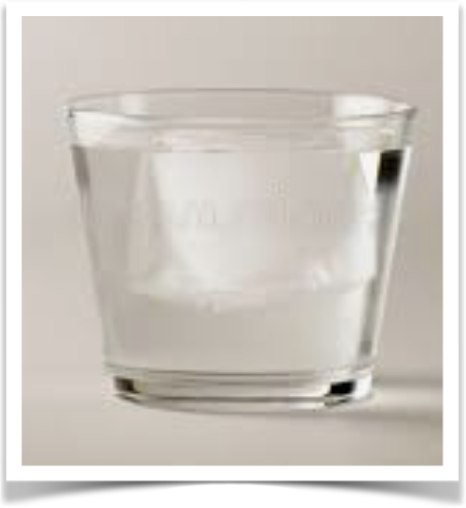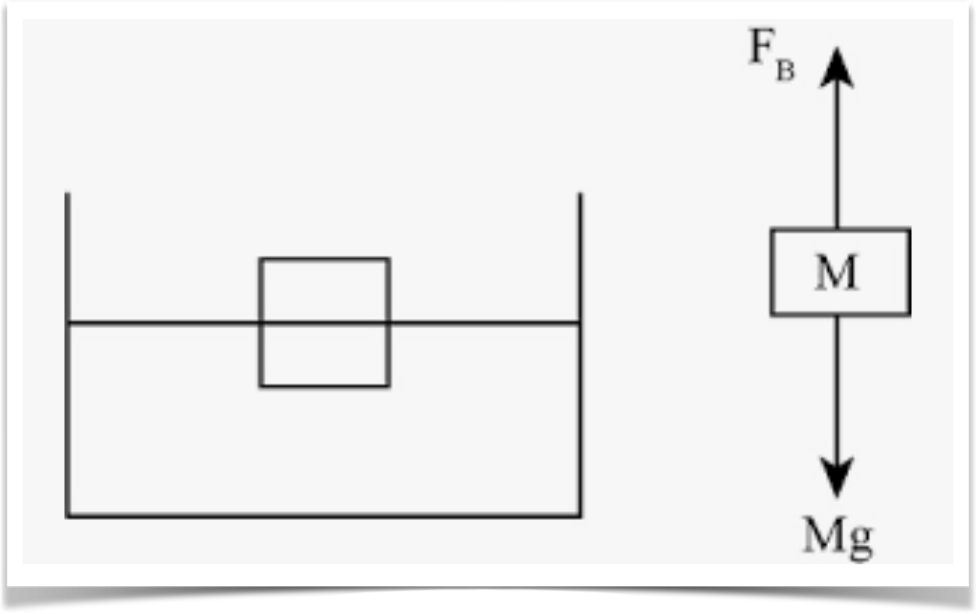# The buoyant force on an object is equal to the weight of the fluid displaced by that object

Archimedes’ principle states “A body wholly or partially immersed in a fluid is buoyed up by a force equal in magnitude to the weight of the fluid displaced by the body.

The volume of an object is equal to its mass divided by density.
Volume = Mass / Density
v = m/d

Following are four applications of buoyancy and Archimedes’ principle. They are:

1. Two identical buckets filled to the brim with water
2. Rock in a boat floating in a pond is tossed into the water
3. About ten percent of a floating iceberg sits above the water
4. Melt an ice cube in a cup of water and the water level remains unchanged

1. Given two identical buckets filled to the brim with water. One bucket has a floating object in it, such as a block of ice or a piece of wood. Both buckets with content weigh the same. The floating object displaces a volume of water equal to its weight.

2. If a rock in a boat floating in a pond is tossed into the water, and the rock sinks, the water level of the pond will drop.

This is because the rock displaces its mass while in the boat, and displaces its volume in the water. This assumes the rock sinks and is therefore more dense than water.

This can be proven mathematically as follows below.

The increase in volume of the pond, when the boat, with a rock in it, is placed in the pond, is equal to the mass of the boat divided by the density of water, plus the mass of the rock divided by the density of water.

The buoyant force on the boat with the rock in it is equal to the weight of the rock and the boat. When the boat, with the rock in it, is floating in the pond, the volume of water displaced is the combined mass of the boat and rock divided by density of water.

The floating boat and with rock displace a volume of water that can be expressed as:

mass of boat                   mass of rock
V1  =   ——————       +     ——————
density of water             density of water

Remember that volume of any material is equal to mass over density

When the rock is in the water, it displaces its volume, not its mass. The volume of water displaced by the submerged rock and floating boat is the mass of the boat divided by the density of water, plus the volume of the rock. The volume of the rock can be expressed as its mass divided by density. The rock is more dense than the water, evidenced by the fact that it sinks.

The volume of water now displaced by the floating boat and rock submerged in water is mass of the boat divided by density of water, plus mass of the rock divided by density of the rock.

The floating boat and submerged rock displace a volume of water that can be expressed as:

mass of boat                   mass of rock
V2 =    ——————       +     ——————
density of water             density of rock

Subtract the second expression from the first and the result is

mass of rock                  mass of rock
V1 - V2 =    ——————       +     ——————
density of water             density of rock

Look at the right side of this equation. On the right hand side of the expression, the mass of rock is numerator in both fractions. Density of rock is larger than density of water, meaning the second fraction is smaller than the first, and the result of the subtraction is a number greater than zero and therefore positive. This means that V1 - V2 is a positive number, which can only be true of V1 is larger than V2. V1 represents the volume of water displaced by the boat with the rock in it, and V2 represents the volume of water displaced by the floating boat and submerged rock, which has now been algebraically proven to be a smaller value. Therefore the water level of the pond goes down when the rock is thrown out of the boat and into the water.

3. Just over ten percent of an iceberg sits above water. This is because ice is less dense than water.

The density of water is about 997 kilograms per cubic meter. Salt water, according to most physics text books, weighs about 1,024 kilograms per cubic meter. When water freezes it squeezes out most of the salt brine and becomes relatively pure frozen water, with a density of about 917 kilograms per cubic meter. Ice floats because it is less dense than water.

The weight of a floating iceberg, or any other floating object, is equal to its density times volume times gravity.

Weight of iceberg = Density of ice * Volume of iceberg * g

The volume of water displaced by a floating object has the same weight as the submerged portion of the floating object. This is equal to the buoyant force on the floating object. The buoyant force is equal to the density of water, times the volume of displaced water, times gravity.

Buoyant force = Density of water * Volume of displaced water * g
Weight of displaced water = Density of water * Volume of displaced water * g

A floating iceberg is in a state of equilibrium. This means the buoyant force is equal to the weight of the iceberg.

Buoyant force = Weight of iceberg

Density of ice * volume of iceberg * g =
Density of water * volume of displaced water * g

cancelling g which exists on both sides leaves

Density of ice * volume of iceberg = Density of water * volume of displaced water

Divide both sides by density of salt water and by volume of iceberg gives

Density of ice/density of water = volume displaced water / volume of iceberg

Density of ice = 917 kg/m^3
Density of salt water = 1024 kg/m^3

Meaning 917/1024 = volume of displaced water / volume of iceberg = 0.896 = 89.6 percent

The volume of water displaced is the volume of the submerged portion of the iceberg, which is 89.6 % of the iceberg, with the remaining 10.4 percent above water.

4. Ice cube floating in a glass of water.Given an ice cube is floating in a glass of water. After the ice cube melts, the water line will remain the same and will not rise or fall.

An ice cube floating in water displaces an amount of water that weighs the same as the ice cube.

The amount of water, aka the volume of water, that is displaced by the ice cube, is equal in mass to the mass of the ice.

A floating object displaces its mass.

A sinking object displaces its volume

In On Floating Bodies, Archimedes wrote, in 246 BC:

“Any object, totally or partially immersed in a fluid or liquid, is buoyed up by a force equal to the weight of the fluid displaced by the object.”

A floating object, such as an ice cube in water and at rest is acted on by an upward, or buoyant force. The magnitude of that upward buoyant force is equal to the weight of the fluid displaced by the body.

The buoyant force on a floating object such as an ice cube is also equivalent in magnitude to the weight of the floating object and is opposite in direction. The ice cube does not rise or sink; it floats. For example, a boat launched into water sinks into the water until the weight of the water that the boat displaces is equal to its own weight.

A free body diagram of the opposing forces on a floating ice cube would show mg (mass times gravity) in the negative-Y direction, opposed by fb (force of buoyancy) in the positive-Y direction.If you could remove a floating ice cube from a cup of water and leave a ‘hole’ in the water where the cube used to float, that hold would take an amount of water equal in weight to that of the ice cube. Melt that ice cube, pour it into the hole, the melted water will exactly fill the hole, and  leave the remaining water undisturbed.

An empirical test produces the expected result: